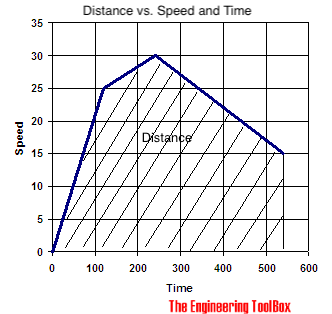Engineering ToolBox - Resources, Tools and Basic Information for Engineering and Design of Technical Applications!

# Distance Traveled vs. Speed and Time - Calculator and Chart

## Velocity plotted in time used diagram.

For a graph with velocity vs. time - the area under the graph is distance traveled:You can use the calculator below to summarize traveled distance:

Distance traveled: (m, ft, km, miles - depending on the units used)

### Example

A car travels intervals with constant speed:

1. 25 m/s in 120 s
2. 30 m/s in 220 s
3. 15 m/s in 300 s

The total distance traveled by the car can be calculated as

(25 m/s) (120 s) + (30 m/s) (220 s) + (15 m/s) (300 s)

= 14100 m

## Related Topics

• ### Dynamics

Motion - velocity and acceleration, forces and torque.
• ### Miscellaneous

Engineering related topics like Beaufort Wind Scale, CE-marking, drawing standards and more.

## Related Documents

• ### Angular Motion - Power and Torque

Angular velocity and acceleration vs. power and torque.
• ### Centripetal and Centrifugal Acceleration Force

Forces due to circular motion and centripetal / centrifugal acceleration.
• ### Dynamic Pressure

Dynamic pressure is the kinetic energy per unit volume of a fluid in movement.
• ### Formulas of Motion - Linear and Circular

Linear and angular (rotation) acceleration, velocity, speed and distance.
• ### Impulse and Impulse Force

Forces acting a very short time are called impulse forces.
• ### Kinetic Energy

Energy possessed by an object's motion is kinetic energy.
• ### Piston Engines - Displacement

Calculate piston engine displacement.
• ### Projectile Range

Calculate the range of a projectile - a motion in two dimensions.
• ### Torque - Work done and Power Transmitted

The work done and power transmitted by a constant torque.

## Engineering ToolBox - SketchUp Extension - Online 3D modeling!

Add standard and customized parametric components - like flange beams, lumbers, piping, stairs and more - to your Sketchup model with the Engineering ToolBox - SketchUp Extension - enabled for use with older versions of the amazing SketchUp Make and the newer "up to date" SketchUp Pro . Add the Engineering ToolBox extension to your SketchUp Make/Pro from the Extension Warehouse !

We don't collect information from our users. More about

## Citation

• The Engineering ToolBox (2008). Distance Traveled vs. Speed and Time - Calculator and Chart. [online] Available at: https://www.engineeringtoolbox.com/speed-time-graph-d_1373.html [Accessed Day Month Year].

Modify the access date according your visit.

9.19.12